HDL Implementation of a Digital Down-Converter for LTE

This example shows how to design a digital down-converter (DDC) for radio communication applications such as LTE, and generate HDL code.

Introduction

DDCs are widely used in digital communication receivers to convert radio frequency (RF) or intermediate frequency (IF) signals to baseband. The DDC operation shifts the signal to a lower frequency and reduces its sampling rate to facilitate subsequent processing stages. The DDC in this example performs complex frequency translation followed by sample rate conversion using a four-stage filter chain. The example starts by designing the DDC with DSP System Toolbox™ functions in floating point. Then, each stage is converted to fixed point, and used in a Simulink® model that generates synthesizable HDL code. The example uses these two test signals to demonstrate and verify the DDC operation:

• A sinusoid that is modulated onto a 32 MHz IF carrier.

• An LTE downlink signal with a bandwidth of 1.4 MHz that is modulated onto a 32 MHz IF carrier.

The example compares the signal quality at the output of the floating-point DDC with the signal quality at the output of the fixed-point DDC.

Finally, the example presents an implementation of the filter chain for FPGAs, and synthesis results.

This example uses DDCTestUtils, a helper class that contains functions for generating stimulus and analyzing the DDC output. For more information, see the DDCTestUtils.m file.

DDC Structure

The DDC consists of a numerically controlled oscillator (NCO), mixer, and decimating filter chain. The filter chain consists of a CIC decimator, CIC gain correction, a CIC compensation decimator (FIR), a halfband FIR decimator, and a final FIR decimator.

The overall response of the filter chain is equivalent to that of a single decimation filter with the same specification. However, splitting the filter into multiple decimation stages results in a more efficient design that uses fewer hardware resources.

The cascade integrator-comb (CIC) decimator provides a large initial decimation factor, which enables subsequent filters to work at lower rates. The CIC compensation decimator improves the spectral response by compensating for the CIC droop while decimating by two. The halfband is an intermediate decimator, and the final decimator implements the precise Fpass and Fstop characteristics of the DDC. The lower sampling rates near the end of the chain mean the later filters can optimize resource use by sharing multipliers.

This figure shows a block diagram of the DDC.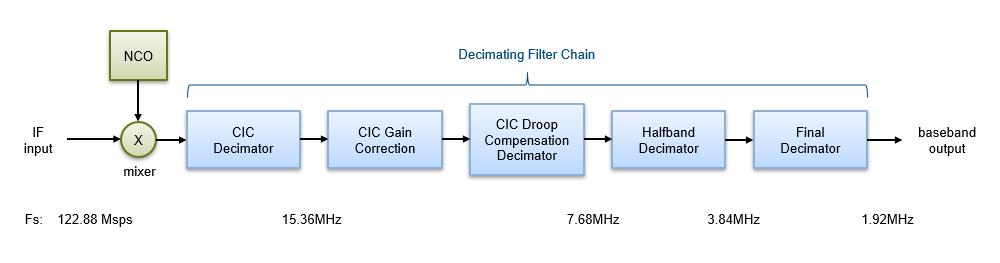The sample rate of the input to the DDC is 122.88 Msps, and the output sample rate is 1.92 Msps. These rates give an overall decimation factor of 64. LTE receivers use 1.92 Msps as the typical sampling rate for cell search and master information block (MIB) recovery. The DDC filters are designed to suit this application. The DDC is optimized to run at a clock rate of 122.88 MHz.

DDC Design

This section explains how to design the DDC using floating-point operations and filter-design functions in MATLAB®.

DDC Parameters

This example designs the DDC filter characteristics to meet these desired response values for the given input sampling rate and carrier frequency.

FsIn = 122.88e6;    % Sampling rate of DDC input
FsOut = 1.92e6;     % Sampling rate of DDC output
Fc = 32e6;          % Carrier frequency
Fpass = 540e3;      % Passband frequency, equivalent to 36x15kHz LTE subcarriers
Fstop = 700e3;      % Stopband frequency
Ap = 0.1;           % Passband ripple
Ast = 60;           % Stopband attenuation

CIC Decimator

The first filter stage is a CIC decimator because of its ability to efficiently implement a large decimation factor. The response of a CIC filter is similar to a cascade of moving average filters, but a CIC filter uses no multiplication or division. As a result,the CIC filter has a large DC gain.

cicParams.DecimationFactor = 8;
cicParams.DifferentialDelay = 1;
cicParams.NumSections = 3;
cicParams.FsOut = FsIn/cicParams.DecimationFactor;

cicFilt = dsp.CICDecimator(cicParams.DecimationFactor, ...
cicParams.DifferentialDelay,cicParams.NumSections) %#ok<*NOPTS>
cicGain = gain(cicFilt)
cicFilt =

dsp.CICDecimator with properties:

DecimationFactor: 8
DifferentialDelay: 1
NumSections: 3
FixedPointDataType: 'Full precision'

cicGain =

512

Because the CIC gain is a power of two, a hardware implementation can easily correct for the gain factor by using a shift operation. For analysis purposes, the example represents the gain correction in MATLAB with a one-tap dsp.FIRFilter System object™.

cicGainCorr = dsp.FIRFilter('Numerator',1/cicGain)
cicGainCorr =

dsp.FIRFilter with properties:

Structure: 'Direct form'
NumeratorSource: 'Property'
Numerator: 0.0020
InitialConditions: 0

Use get to show all properties

Display the magnitude response of the CIC filter with and without gain correction by using fvtool. For analysis, combine the CIC filter and the gain correction filter into a dsp.FilterCascade System object. CIC filters use fixed-point arithmetic internally, so fvtool plots both the quantized and unquantized responses.

ddcPlots.cicDecim = fvtool(...
cicFilt, ...
'Fs',[FsIn,FsIn]);
DDCTestUtils.setPlotNameAndTitle('CIC Decimator');
legend(...
'CIC No Correction: Quantized', ...
'CIC No Correction: Reference', ...
'CIC With Gain Correction: Quantized', ...
'CIC With gain correction: Reference');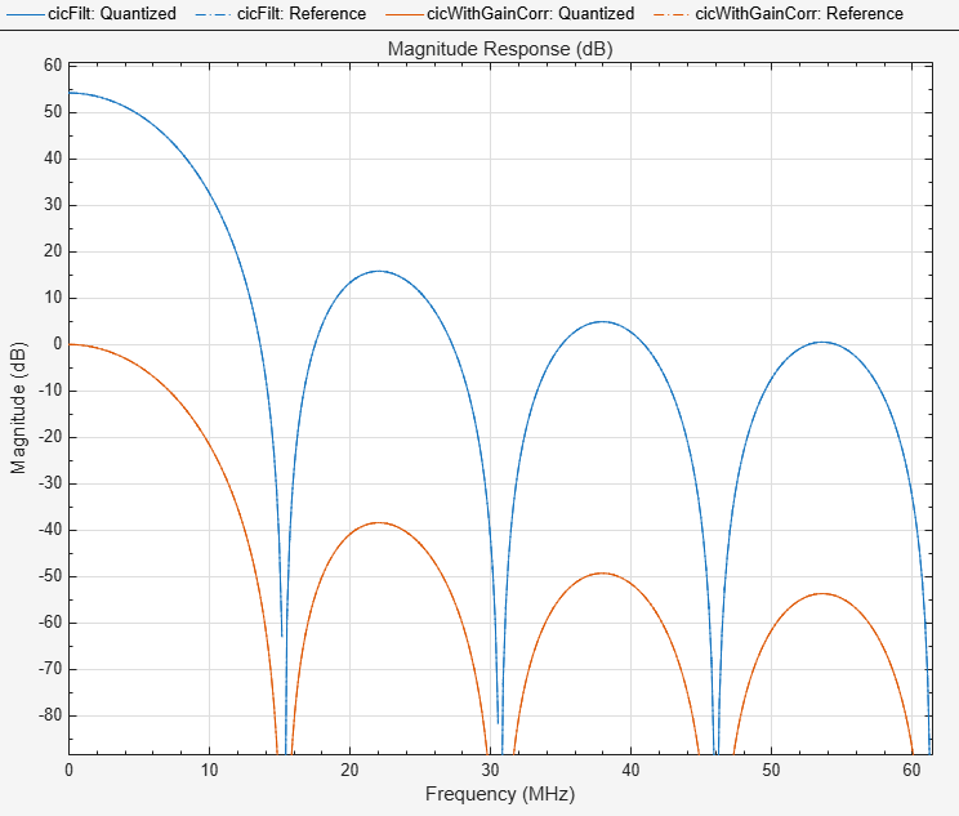CIC Droop Compensation Filter

Because the magnitude response of the CIC filter has a significant droop within the passband region, the example uses a FIR-based droop compensation filter to flatten the passband response. The droop compensator has the same properties as the CIC decimator. This filter implements decimation by a factor of two, so you must also specify bandlimiting characteristics for the filter. Use the design function to return a filter System object with the specified characteristics.

compParams.R = 2;                                % CIC compensation decimation factor
compParams.Fpass = Fstop;                        % CIC compensation passband frequency
compParams.FsOut = cicParams.FsOut/compParams.R; % New sampling rate
compParams.Fstop = compParams.FsOut - Fstop;     % CIC compensation stopband frequency
compParams.Ap = Ap;                              % Same passband ripple as overall filter
compParams.Ast = Ast;                            % Same stopband attenuation as overall filter

compSpec = fdesign.decimator(compParams.R,'ciccomp', ...
cicParams.DifferentialDelay, ...
cicParams.NumSections, ...
cicParams.DecimationFactor, ...
'Fp,Fst,Ap,Ast', ...
compParams.Fpass,compParams.Fstop,compParams.Ap,compParams.Ast, ...
cicParams.FsOut);
compFilt = design(compSpec,'SystemObject',true)
compFilt =

dsp.FIRDecimator with properties:

DecimationFactor: 2
NumeratorSource: 'Property'
Numerator: [-0.0398 -0.0126 0.2901 0.5258 0.2901 -0.0126 -0.0398]
Structure: 'Direct form'

Use get to show all properties

Plot the combined response of the CIC filter (with gain correction) and droop compensation.

ddcPlots.cicComp = fvtool(...
'Fs',FsIn,'Legend','off');
DDCTestUtils.setPlotNameAndTitle('CIC Decimation and Droop Compensation');Halfband Decimator

The halfband filter provides efficient decimation by two. Halfband filters are efficient because approximately half of their coefficients are equal to zero, and those multipliers are excluded from the hardware implementation.

hbParams.FsOut = compParams.FsOut/2;
hbParams.TransitionWidth = hbParams.FsOut - 2*Fstop;
hbParams.StopbandAttenuation = Ast;

hbSpec = fdesign.decimator(2,'halfband',...
'Tw,Ast', ...
hbParams.TransitionWidth, ...
hbParams.StopbandAttenuation, ...
compParams.FsOut);
hbFilt = design(hbSpec,'SystemObject',true)
hbFilt =

dsp.FIRDecimator with properties:

DecimationFactor: 2
NumeratorSource: 'Property'
Numerator: [0.0089 0 -0.0565 0 0.2977 0.5000 0.2977 0 -0.0565 ... ]
Structure: 'Direct form'

Use get to show all properties

Plot the response of the DDC up to the halfband filter output.

ddcPlots.halfbandFIR = fvtool(...
'Fs',FsIn,'Legend','off');
DDCTestUtils.setPlotNameAndTitle('CIC Decimation, Droop Compensation, and Halfband FIR');Final FIR Decimator

The final FIR implements the detailed passband and stopband characteristics of the DDC. This filter has more coefficients than the earlier FIR filters, but because it operates at a lower sampling rate it can use resource sharing for an efficient hardware implementation.

Add 3 dB of headroom to the stopband attenuation so that the DDC still meets the specification after fixed-point quantization. This value was found empirically by using fvtool.

finalSpec = fdesign.decimator(2,'lowpass', ...
'Fp,Fst,Ap,Ast',Fpass,Fstop,Ap,Ast+3,hbParams.FsOut);
finalFilt = design(finalSpec,'equiripple','SystemObject',true)
finalFilt =

dsp.FIRDecimator with properties:

DecimationFactor: 2
NumeratorSource: 'Property'
Numerator: [9.3365e-04 0.0013 9.3466e-04 -5.3189e-04 -0.0022 ... ]
Structure: 'Direct form'

Use get to show all properties

Visualize the overall magnitude response of the DDC.

compFilt,hbFilt,finalFilt);
ddcPlots.overallResponse = fvtool(ddcFilterChain, ...
'Fs',FsIn,'Legend','off');
DDCTestUtils.setPlotNameAndTitle('Overall DDC Filter Chain');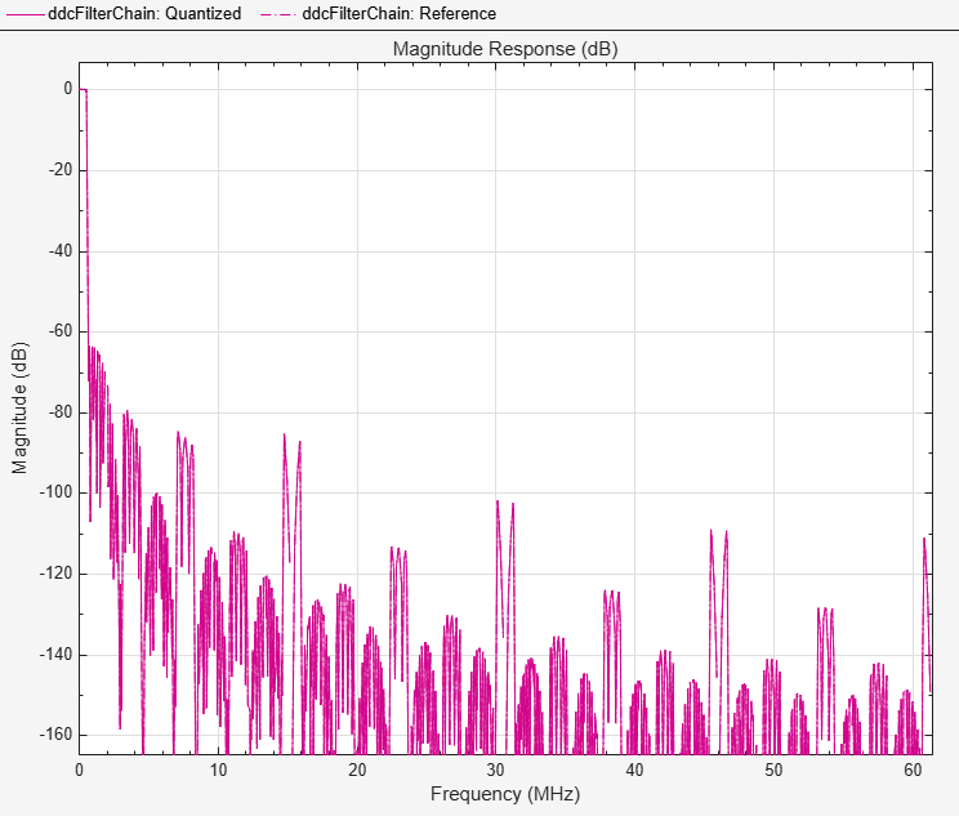Fixed-Point Conversion

The frequency response of the floating-point DDC filter chain now meets the specification. Next, quantize each filter stage to use fixed-point types and analyze them to confirm that the filter chain still meets the specification.

Filter Quantization

This example uses 16-bit coefficients, which are sufficient to meet the specification. Using fewer than 18 bits for the coefficients minimizes the number of DSP blocks that are required for an FPGA implementation. The input to the DDC filter chain is 16-bit data with 15 fractional bits. The filter outputs are 18-bit values, which provide extra headroom and precision in the intermediate signals.

For the CIC decimator, choosing the 'Minimum section word lengths' fixed-point data type option automatically optimizes the internal wordlengths based on the output wordlength and other CIC parameters.

cicFilt.FixedPointDataType = 'Minimum section word lengths';
cicFilt.OutputWordLength = 18;

Configure the fixed-point properties of the gain correction and FIR-based System objects. The object uses the default RoundingMethod and OverflowAction property values ('Floor' and 'Wrap' respectively).

% CIC Gain Correction
cicGainCorr.FullPrecisionOverride = false;
cicGainCorr.CoefficientsDataType = 'Custom';
cicGainCorr.CustomCoefficientsDataType = numerictype(fi(cicGainCorr.Numerator,1,16));
cicGainCorr.OutputDataType = 'Custom';
cicGainCorr.CustomOutputDataType = numerictype(1,18,16);

% CIC Droop Compensation
compFilt.FullPrecisionOverride = false;
compFilt.CoefficientsDataType = 'Custom';
compFilt.CustomCoefficientsDataType = numerictype([],16,15);
compFilt.ProductDataType = 'Full precision';
compFilt.AccumulatorDataType = 'Full precision';
compFilt.OutputDataType = 'Custom';
compFilt.CustomOutputDataType = numerictype([],18,16);

% Halfband
hbFilt.FullPrecisionOverride = false;
hbFilt.CoefficientsDataType = 'Custom';
hbFilt.CustomCoefficientsDataType = numerictype([],16,15);
hbFilt.ProductDataType = 'Full precision';
hbFilt.AccumulatorDataType = 'Full precision';
hbFilt.OutputDataType = 'Custom';
hbFilt.CustomOutputDataType = numerictype([],18,16);

% FIR
finalFilt.FullPrecisionOverride = false;
finalFilt.CoefficientsDataType = 'Custom';
finalFilt.CustomCoefficientsDataType = numerictype([],16,15);
finalFilt.ProductDataType = 'Full precision';
finalFilt.AccumulatorDataType = 'Full precision';
finalFilt.OutputDataType = 'Custom';
finalFilt.CustomOutputDataType = numerictype([],18,16);

Fixed-Point Analysis

Inspect the quantization effects with fvtool. You can analyze the filters individually or in a cascade. fvtool shows the quantized and unquantized (reference) responses overlayed. For example, this figure shows the effect of quantizing the final FIR filter stage.

ddcPlots.quantizedFIR = fvtool(finalFilt, ...
'Fs',hbParams.FsOut,'arithmetic','fixed');
DDCTestUtils.setPlotNameAndTitle('Quantized Final Filter');
legend('quantized','reference');Redefine the ddcFilterChain cascade object to include the fixed-point properties of the individual filters. Then, use fvtool to analyze the entire filter chain and confirm that the quantized DDC still meets the specification.

cicGainCorr,compFilt,hbFilt,finalFilt);
ddcPlots.quantizedDDCResponse = fvtool(ddcFilterChain, ...
'Fs',FsIn,'Arithmetic','fixed');
DDCTestUtils.setPlotNameAndTitle('Quantized DDC Filter Chain');
legend('quantized','reference');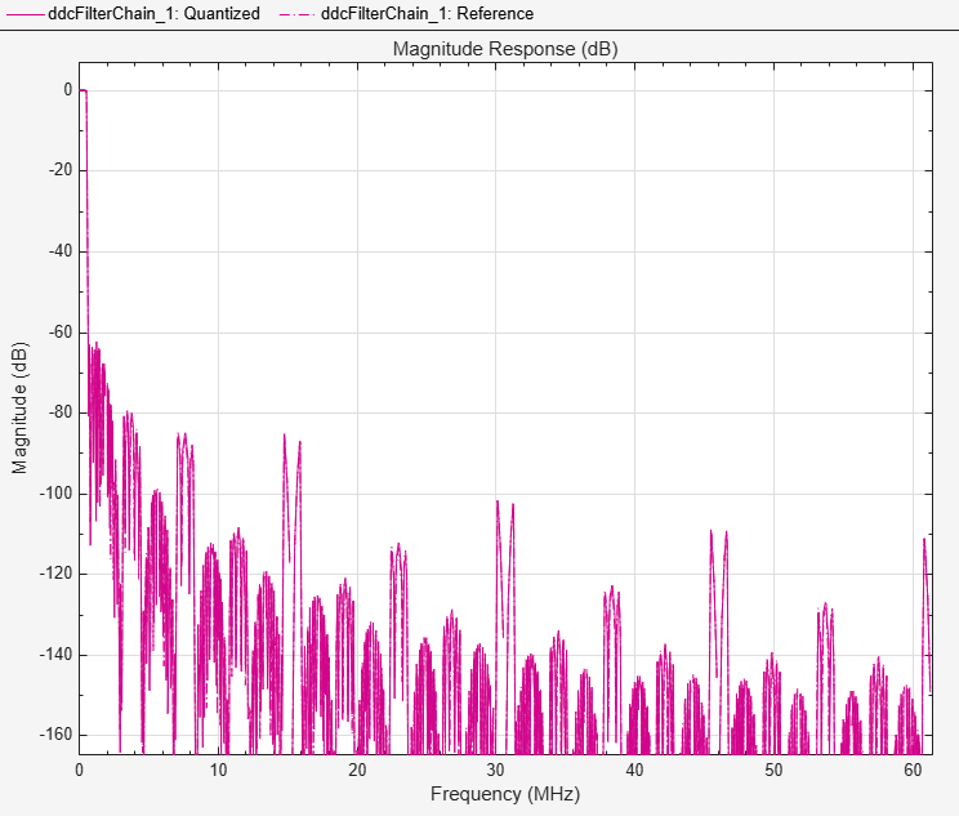The next step in the design flow is to implement the DDC in Simulink using blocks that support HDL code generation.

Model Configuration

The model relies on variables in the MATLAB workspace to configure the blocks and settings. It uses the same filter chain variables defined earlier in the example. Next, define the NCO characteristics and the input signal. The example uses these characteristics to configure the NCO block.

Specify the desired frequency resolution. Calculate the number of accumulator bits that are required to achieve the desired resolution, and then define the number of quantized accumulator bits. The NCO uses the quantized output of the accumulator to address the sine lookup table. Also compute the phase increment the NCO must use to generate the specified carrier frequency. The NCO applies phase dither to those accumulator bits that are removed during quantization.

nco.Fd = 1;
nco.AccWL = nextpow2(FsIn/nco.Fd)+1;
nco.QuantAccWL = 12;
nco.PhaseInc = round((-Fc*2^nco.AccWL)/FsIn);
nco.NumDitherBits = nco.AccWL-nco.QuantAccWL;

The input to the DDC comes from the ddcIn variable. For now, assign a dummy value for ddcIn so that the model can compute its data types. During testing, ddcIn provides input data to the model.

ddcIn = 0; %#ok<NASGU>

Model Structure

This figure shows the top level of the DDC Simulink model. The model imports the ddcIn variable from the MATLAB workspace by using a Signal From Workspace block, converts the input signal to 16-bit values, and applies the signal to the DDC. You can generate HDL code from the HDL_DDC subsystem.

modelName = 'DDCHDLImplementation';
open_system(modelName);
set_param(modelName,'SimulationCommand','Update');
set_param(modelName,'Open','on');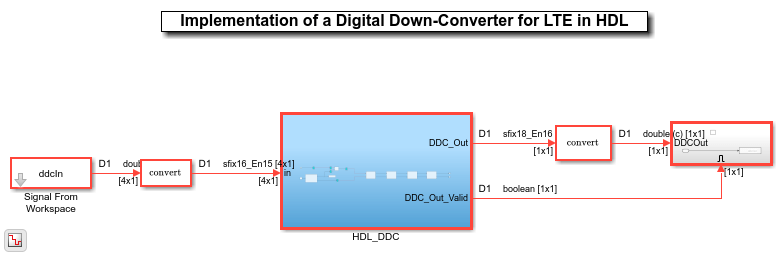The DDC implementation is inside the HDL_DDC subsystem. The NCO HDL Optimized block generates a complex phasor at the carrier frequency. This signal goes to a mixer that multiplies the phasor with the input signal. Then, the output of the mixer is passed to the filter chain and decimated to 1.92 Msps.

set_param([modelName '/HDL_DDC'],'Open','on');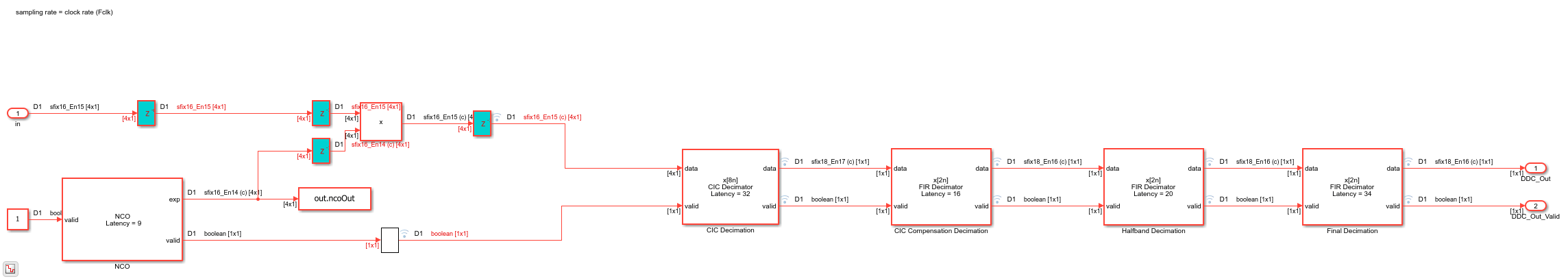NCO Block Parameters

The NCO block in the model is configured with the parameters defined in the nco structure. This figure shows both tabs of the NCO HDL Optimized block parameters dialog.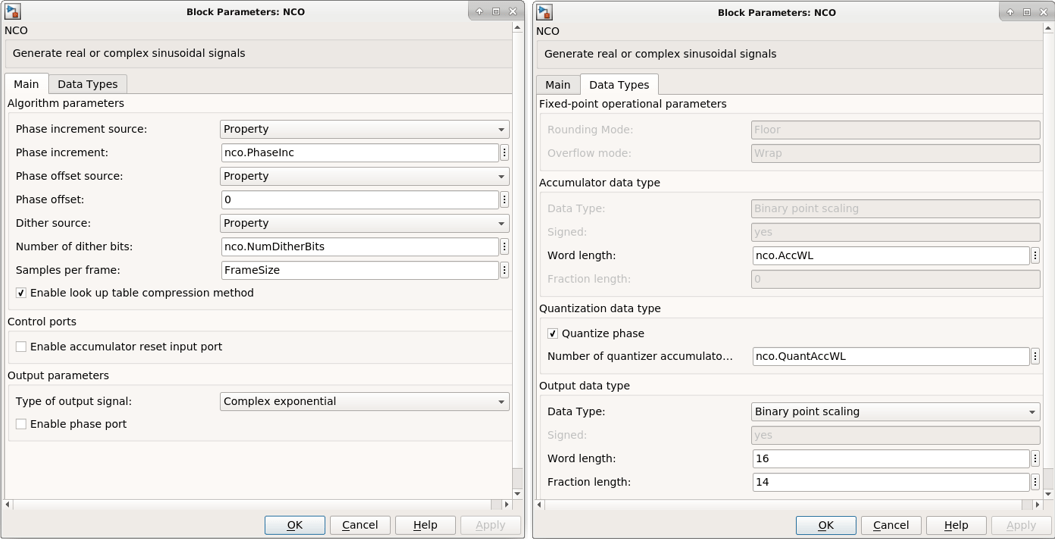CIC Decimation and Gain Correction

The first filter stage is a CIC Decimator that is implemented with a CIC Decimation HDL Optimized block. The block parameters are set to the cicParams structure values. To implement the gain correction, the model selects the Gain correction parameter.

The model configures the filters by using the properties of the corresponding System objects. The CIC compensation, halfband decimation, and final cecimation filters operate at effective sample rates that are lower than the clock rate by factors of 8, 16, and 32, respectively. The model implements these sample rates by using the valid input signal to indicate which samples are valid at each rate. The signals in the filter chain all have the same Simulink sample time.

The CIC compensation, halfband decimation, and final decimation filters are each implemented by a MATLAB Function block and two Discrete FIR Filter HDL Optimized blocks in a polyphase decomposition. Polyphase decomposition implements the transform function, where,, and. Polyphase decomposition is a resource-efficient way to implement decimation filters. The MATLAB Function block holds two input samples every two cycles and passes them at the same time to the parallel pair of Discrete FIR Filter HDL Optimized blocks,and. The lower subfilter,, and the upper subfilter,, each contain half of the filter coefficients and process half of the input data.

For example, the CIC Compensation Decimation subsystem implements a seven-coefficient filter. The upper subfilter,, has four coefficients and the lower subfilter,, has three coefficients. Each filter receives a sample and generates an output every 16 cycles. Because each filter processes one sample every 16 cycles, the subfilter blocks can share hardware resources in time. To optimize hardware resources in this way, both of the subfilters have the Filter structure parameter set to Partly serial systolic.

This figure shows the CIC Compensation Decimation subsystem. The Halfband Decimation and Final Decimation subsystems use the same structure.The model configures all the filter blocks by using the parameters defined in their corresponding structures. For example, this figure shows the block parameters for the CIC Compensation Decimation block. The Number of cycles parameter is the minimum number of cycles between input samples. The sample rate of the input to the CIC Compensation Decimation block is cicParams.DecimationFactor*compParams.R, which is one sample every 16 cycles for each subfilter.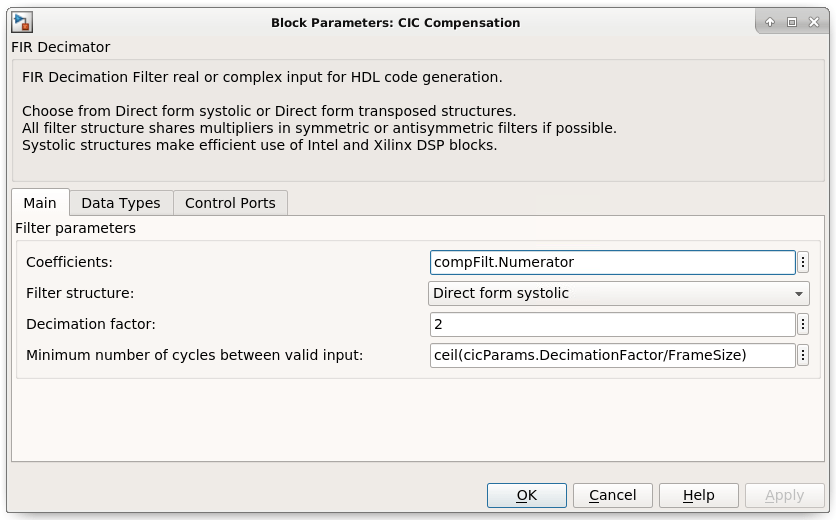The serial filter implementation reuses the multipliers in time over the number of clock cycles you specify. Without this optimization, the CIC Compensation Decimation subsystem with complex input data uses 14 multipliers. After the optimization,andeach use use two multipliers for a total of four. Similarly, the Halfband Decimation and Final Decimation subsystems use four multipliers each.

Sinusoid on Carrier Test and Verification

To test the DDC, modulate a 40 kHz sinusoid onto the carrier frequency and pass the modulated sine wave through the DDC. Then, measure the spurious free dynamic range (SFDR) of the resulting tone and the SFDR of the NCO output. Plot the SFDR of the NCO and the fixed-point DDC output.

% Initialize random seed before executing any simulations.
rng(0);

% Generate a 40 kHz test tone, modulated onto the carrier.
ddcIn = DDCTestUtils.GenerateTestTone(40e3,Fc);

% Demodulate the test signal with the floating-point DDC.
ddcOut = DDCTestUtils.DownConvert(ddcIn,FsIn,Fc,ddcFilterChain);
release(ddcFilterChain);

% Demodulate the test signal by running the Simulink model.
out = sim(modelName);

% Measure the SFDR of the NCO, floating-point DDC outputs, and fixed-point
% DDC outputs.
results.sfdrNCO = sfdr(real(out.ncoOut),FsIn);
results.sfdrFloatDDC = sfdr(real(ddcOut),FsOut);
results.sfdrFixedDDC = sfdr(real(out.ddcOut),FsOut);

disp('SFDR Measurements');
disp(['   Floating-point DDC SFDR: ',num2str(results.sfdrFloatDDC) ' dB']);
disp(['   Fixed-point NCO SFDR: ',num2str(results.sfdrNCO) ' dB']);
disp(['   Optimized fixed-point DDC SFDR: ',num2str(results.sfdrFixedDDC) ' dB']);
fprintf(newline);

% Plot the SFDR of the NCO and fixed-point DDC outputs.
ddcPlots.ncoOutSDFR = figure;
sfdr(real(out.ncoOut),FsIn);
DDCTestUtils.setPlotNameAndTitle(['NCO Output Signal ' get(gca,'Title').String]);

ddcPlots.OptddcOutSFDR = figure;
sfdr(real(out.ddcOut),FsOut);
DDCTestUtils.setPlotNameAndTitle(['Optimized Fixed-Point DDC Output Signal ' get(gca,'Title').String]);
SFDR Measurements
Floating-point DDC SFDR: 291.3685 dB
Fixed-point NCO SFDR: 83.0249 dB
Optimized fixed-point DDC SFDR: 108.8419 dB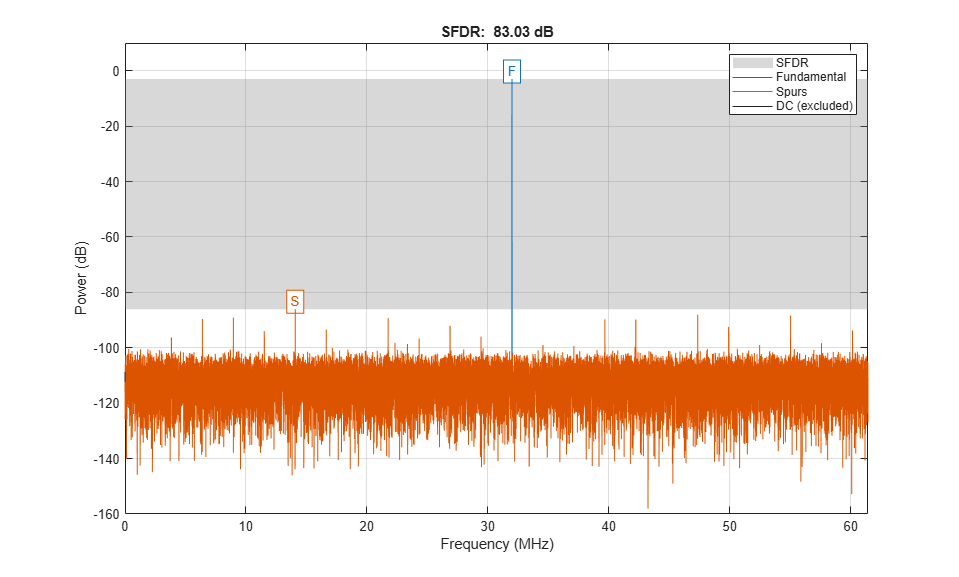LTE Signal Test

You can use an LTE test signal to perform more rigorous testing of the DDC. Generate a standard compliant LTE waveform by using LTE Toolbox™ functions. Then, downconvert the waveform with the DDC model. Use LTE Toolbox functions to measure the error vector magnitude (EVM) of the resulting signals.

rng(0);
% Execute this test only if you have the LTE Toolbox product.

% Generate a modulated LTE test signal by using the LTE Toolbox functions.
[ddcIn,sigInfo] = DDCTestUtils.GenerateLTETestSignal(Fc);

% Downconvert the signal with the floating-point DDC.
ddcOut = DDCTestUtils.DownConvert(ddcIn,FsIn,Fc,ddcFilterChain);
release(ddcFilterChain);

% Downconvert the signal with the Simulink model.
% Pad the input with zeros to represent propagation latency and return the complete result.
ddcIn = [ddcIn;zeros(320,1)];
out = sim(modelName);

results.evmFloat = DDCTestUtils.MeasureEVM(sigInfo,ddcOut);
results.evmFixed = DDCTestUtils.MeasureEVM(sigInfo,out.ddcOut);

disp('LTE Error Vector Magnitude (EVM) Measurements');
disp(['   Floating-point DDC RMS EVM: '  num2str(results.evmFloat.RMS*100,3) '%']);
disp(['   Floating-point DDC Peak EVM: ' num2str(results.evmFloat.Peak*100,3) '%']);
disp(['   Fixed-point HDL-optimized DDC RMS EVM: '     num2str(results.evmFixed.RMS*100,3) '%']);
disp(['   Fixed-point HDL-optimized DDC Peak EVM: '    num2str(results.evmFixed.Peak*100,3) '%']);
fprintf(newline);

end
LTE Error Vector Magnitude (EVM) Measurements
Floating-point DDC RMS EVM: 0.633%
Floating-point DDC Peak EVM: 2.44%
Fixed-point HDL-optimized DDC RMS EVM: 0.731%
Fixed-point HDL-optimized DDC Peak EVM: 2.69%

HDL Code Generation and FPGA Implementation

To generate the HDL code for this example you must have the HDL Coder™ product. Use the makehdl and makehdltb commands to generate HDL code and an HDL test bench for the HDL_DDC subsystem. The DDC was synthesized on a Xilinx® Zynq®-7000 ZC706 evaluation board. The table shows the post place-and-route resource utilization results. The design met timing with a clock frequency of 313 MHz.

T = table(...
categorical({'LUT'; 'LUTRAM'; 'FF'; 'BRAM'; 'DSP'}), ...
categorical({'2660'; '318'; '5951'; '1.0'; '18'}), ...
'VariableNames',{'Resource','Usage'})
T =

5x2 table

Resource    Usage
________    _____

LUT        2660
LUTRAM     318
FF         5951
BRAM       1.0
DSP        18

DSP System Toolbox DocumentationGet trial now